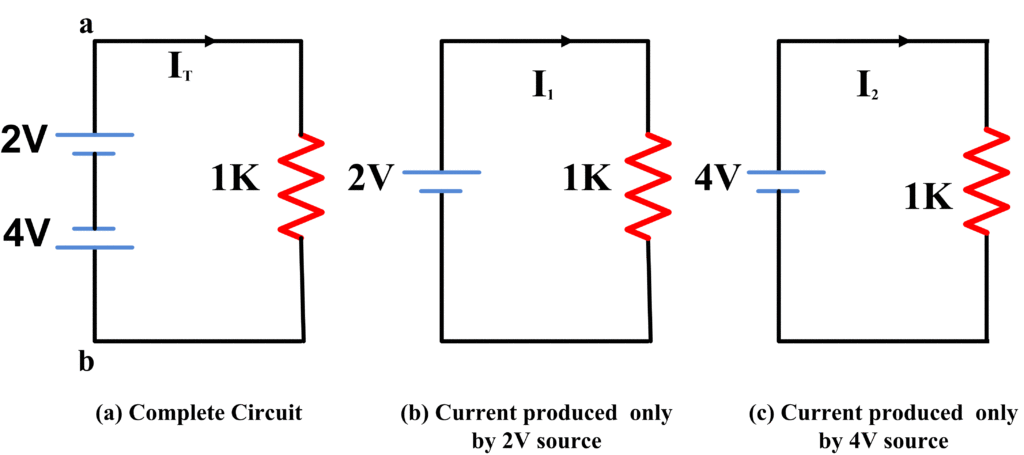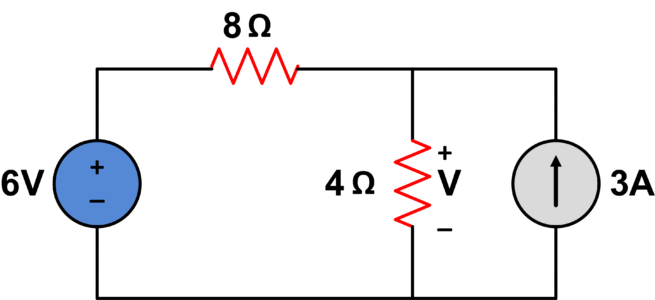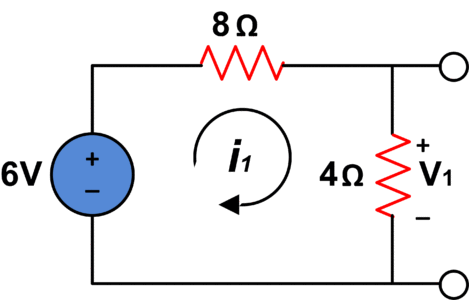# Superposition Theorem

Want create site? Find Free WordPress Themes and plugins.

Superposition theorem is stated as follows:

The current is any circuit element or voltage across any element of a linear bilateral network is the algebraic sum of the currents or voltages separately produced by each source of energy.

Simultaneous equations may be avoided in the solution of a complex network by the application of the superposition theorem. As the effect of each source is considered, the other sources are removed from the circuit. Thus when a voltage source is removed, ideally a short circuit remains. When a current source is removed, ideally an open circuit remains.

Finally, after each source is considered the total voltage or current for an element is found by algebraically adding the components (current or voltage) because of each independent source acting alone.The circuit of Fig. (a) Is a combination of voltage sources of circuit b and c. The total voltage between terminals a and b of circuit a is 6V.Therefore, the total current flowing in circuit a is

${{I}_{T}}=\frac{6V}{1k\Omega }=6mA$

The current flowing in circuit b and c is

${{I}_{1}}=\frac{2V}{1k\Omega }=2mA$

${{I}_{2}}=\frac{4V}{1k\Omega }=4mA$

The addition of I1 and I2 also yields 6mA. The result is not coincidental.

Superposition theorem can be applied to any passive network containing any number of voltage sources, current sources or a combination of both. These sources do not necessarily have to be in series or parallel and in more elaborate networks, they are usually arbitrarily distributed.

It must be stressed that superposition holds only for the linear bilaterally network, where there is a linear relationship between voltage, current, and resistance. Since power loss in the resistor results from the squared relation, as

$P={{I}^{2}}R$

So, superposition cannot be used to calculate power directly, inside the circuit, because of the non-linear nature of power but must be calculated from a total voltage and current value.

## Example of Superposition Theorem

Find V in the circuit given below:After turning off current source, we haveUsing voltage divider;

${{V}_{1}}=\frac{4}{4+8}*6=2V$

Now, turning off the voltage source would give us the following result;Using current divider;

${{i}_{3}}=\frac{8}{4+8}*3=2A$

Whereas;

${{V}_{2}}=4*{{i}_{3}}=4*2=8V$

The algebraic sum of contribution from all sources would be;

$V={{V}_{1}}+{{V}_{2}}=2+8$

$V=10V$

## Summary of Superposition theorem

Superposition theorem can be implemented using the following steps:

1. Draw circuit with all independent sources turned off except one:
• Replace an independent voltage source with a short circuit.
• Replace independent current source with an open circuit.
1. Solve the resultant circuit to find voltage or current using any circuit technique
2. Repeat step 1 for each independent source
3. Take the algebraic sum of all contributions due to independent sources to obtain final voltage or current.
Did you find apk for android? You can find new Free Android Games and apps.